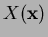Next: A Study in Contrast: Up: A Note on Natural Previous: Governing Equations and Weak

# Implementation of the Natural Element Method (NEM)

The implementation of the Natural Element Method (NEM) by means of a Galerkin-based procedure is parallel to that adopted in FEM or Element-Free Galerkin (EFG) method , with the key distinction that separates the three is in the construction of the shape functionsand their derivatives.

A computational procedure to evaluate the shape functions (n-n coordinates) is outlined in , which is extended by  to compute the derivatives of the interpolating function. In the application of n-n interpolation to PDEs (fluid-structure interaction),  adopted Lasserre's recursive formula  to compute the area (volume of a convex polyhedral in) of the second-order Voronoi cells. The shape functionsas well as their derivativesare computed. In , it is pointed out that Lasserre's formula  is more robust than the one due to , which breaks-down if the pointlies along the edge of a Delaunay triangle. Expressions for the shape functions and their derivatives are provided [3,16,9,10].Next: A Study in Contrast: Up: A Note on Natural Previous: Governing Equations and Weak
N. Sukumar